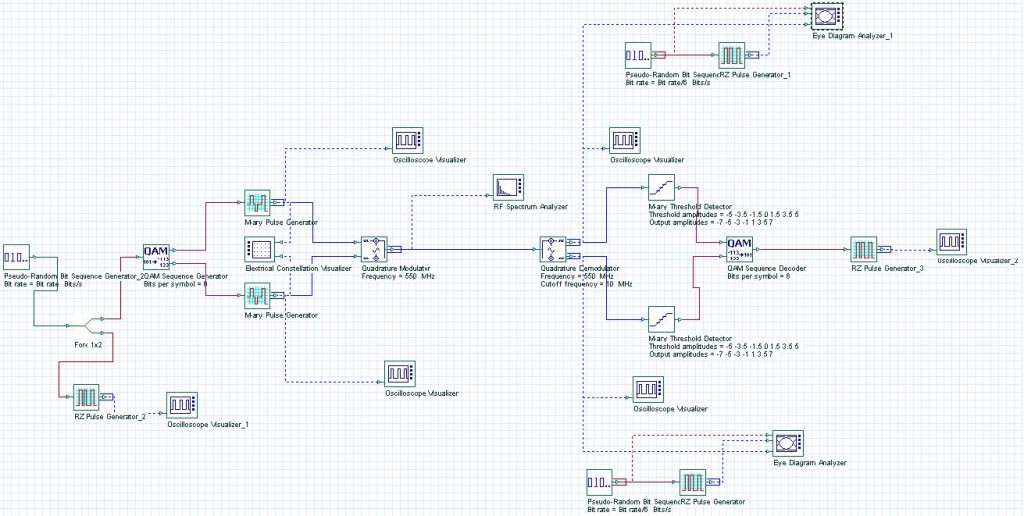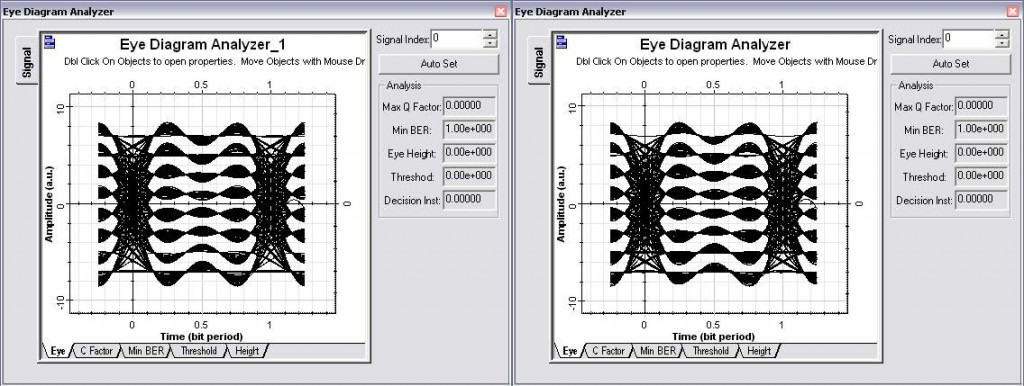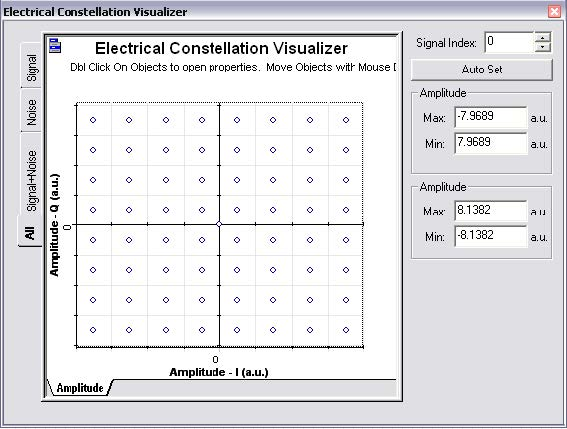## Introduction

The QAM modulation is typically used in CATV system for cable modem transmission. The project file The project file “QAM – Transmitter and Receiver.osd” has an QAM transmitter and receiver (Figure 1) using a 64 QAM transmission (6 bits per symbol).Figure 1: 64 QAM System

The main difference from the DPSK project is the bits per symbol (6), the threshold and output amplitudes for the detector (-7, -5, -3, -1, 1, 3, 5 and 7). Also the global sequence length is 2048.

## Simulation Results

After running the simulation you can visualize the results from the Eye diagrams (Figure 2). Additionally you can visualize the constellation diagram (Figure 3), with the expected results for the In-phase and quadrature points for a 64 QAM modulation scheme.Figure 2: 64 QAM Eye diagrams: In-phase and In-quadrature signalsFigure 3: Constellation diagram for a 64 QAM modulation (6 bits per symbol)